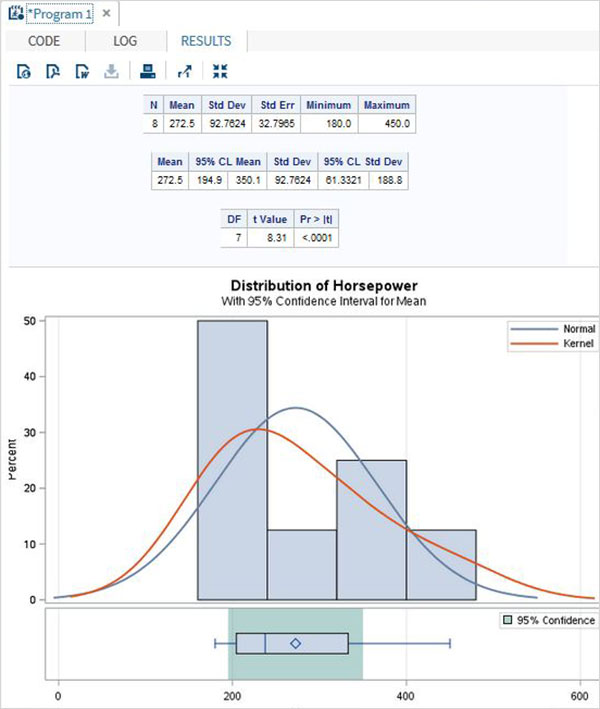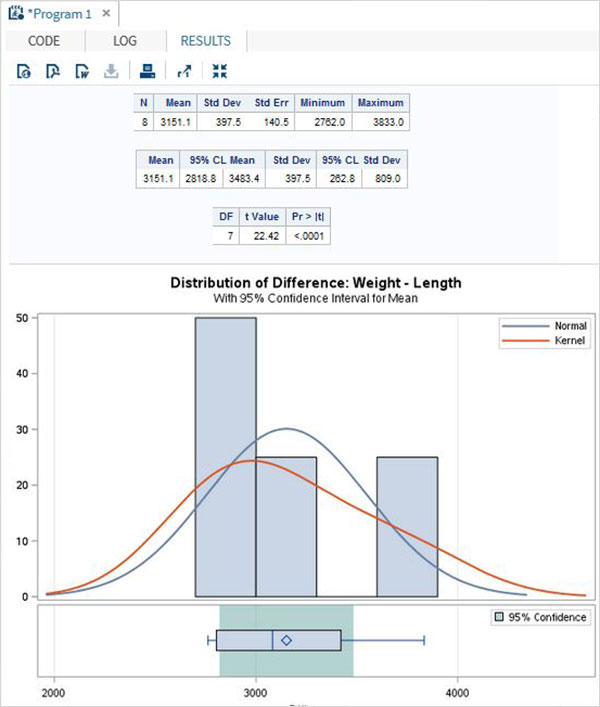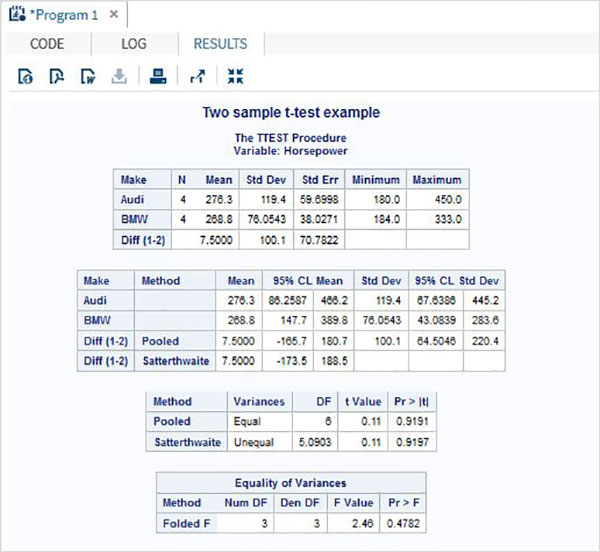# SAS - T Tests

The T-tests are performed to compute the confidence limits for one sample or two independent samples by comparing their means and mean differences. The SAS procedure named PROC TTEST is used to carry out t tests on a single variable and pair of variables.

### Syntax

The basic syntax for applying PROC TTEST in SAS is −

```PROC TTEST DATA = dataset;
VAR variable;
CLASS Variable;
PAIRED Variable_1 * Variable_2;
```

Following is the description of the parameters used −

• Dataset is the name of the dataset.

• Variable_1 and Variable_2 are the variable names of the dataset used in t test.

### Example

Below we see one sample t test in which find the t test estimation for the variable horsepower with 95 percent confidence limits.

```PROC SQL;
create table CARS1 as
SELECT make, type, invoice, horsepower, length, weight
FROM
SASHELP.CARS
WHERE make in ('Audi','BMW')
;
RUN;

proc ttest data = cars1 alpha = 0.05 h0 = 0;
var horsepower;
run;
```

When the above code is executed, we get the following result −## Paired T-test

The paired T Test is carried out to test if two dependent variables are statistically different from each other or not.

### Example

As length and weight of a car will be dependent on each other we apply the paired T test as shown below.

```proc ttest data = cars1 ;
paired weight*length;
run;
```

When the above code is executed, we get the following result −### Two sample t-test

This t-test is designed to compare means of same variable between two groups.

### Example

In our case we compare the mean of the variable horsepower between the two different makes of the cars("Audi" and "BMW").

```proc ttest data = cars1 sides = 2 alpha = 0.05 h0 = 0;
title "Two sample t-test example";
class make;
var horsepower;
run;
```

When the above code is executed, we get the following result −